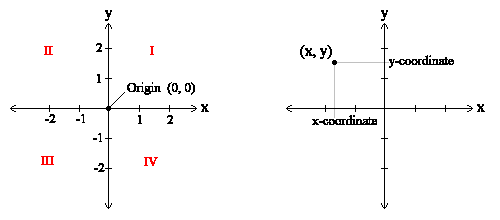Problems
220 - Midpoint

Midpoint

Time Limit: 1 sec

The Problem

• Any point in 2-D may be located by an ordered pair (x, y).
• The x-coordinate, x, is the value on the x-axis vertically above or below the point.
• The y-coordinate, y, is the value on the y-axis horizontally right or left of the point.

Make a simple program that reads 4 variables named x1, y1 and x2, y2 which are represent two points (x1, y1) and (x2, y2). You task is to find the midpoint between 2 given points (x1, y1) and(x2, y2).The Input

The input file contains 4 integer numbers x1, y1x2, and y2 (x1, y1x2, y2 < 1000).

The Output

Output will show two intiger values seperated by space which represend the midpoint in a separate line.

Sample Input

480 785 472 233

Sample Output

476 509

Problem Setter: Shahin ShamS